# Aquarium II

Calculate how much glass we need to build an aquarium with a rectangular shape with base 70 cm × 70 cm and a height of 70 cm, if the waste is 2%. Aquarium haven't top glass.

Result

x =  2.5 m2

#### Solution:

$x = 1.02 \cdot (0.7 \cdot 0.7 + 2\cdot0.7\cdot0.7 + 2\cdot0.7\cdot0.7) = 2.5 \ m^2$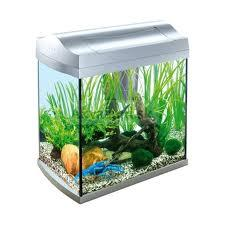Our examples were largely sent or created by pupils and students themselves. Therefore, we would be pleased if you could send us any errors you found, spelling mistakes, or rephasing the example. Thank you!

Leave us a comment of this math problem and its solution (i.e. if it is still somewhat unclear...):Be the first to comment!Tips to related online calculators
Do you want to convert area units?

## Next similar math problems:

1. Glass aquarium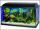How many m2 of glass are needed to produce aquarium with bottom dimensions of 70 cm x 40 cm and 50 cm high?
2. AquariumAquarium is rectangular box with square base containing 76 liters of water. Length of base edge is 42 cm. To what height the water level goes?
3. Surface areaCalculate the surface area of a four-sides 2-m high prism which base is a rectangle with sides 17 cm and 1.3 dm
4. Area of trapezoidThe trapezoid bases are and 7 dm and 11 cm. His height is 4 cm. Calculate the area of trapezoid.
5. AreaCalculate: ?
6. RapeThe agricultural cooperative harvested 525 ares of rape, of which received 5.6 tons of rape seeds. Calculate the yield per hectare of rape.
7. Colza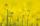In the agricultural cooperative harvested 525 ares of colza, of which received 5.6 tons of seeds. Calculate the yield per hectare.
8. Metal box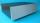How much metal we need for the production of box with dimensions 5 dm, 30 cm and a height of 1 m? Add 12% of the waste and folds.
9. CardboardHow many m2 of cardboard are needed to make the cuboid with dimensions 40 cm 60 cm and 20 cm?
10. Cage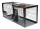How many m2 of mesh farmer use for fencing of a cuboid cage with dimensions 25m, 18m, and 2.5m?
11. Sheet of paperIs the sheet of paper measuring 18 and 25 cm enough for taping 9 cm cubes with an edge? (1 = yes, 0 = no)
12. One hectareHow many square meters are one hectare?
13. Water depthHow many square meters of the inside of the pool wet the water if the pool is 25 meters long and 10 meters wide and the water depth is 1.2 meters everywhere.
14. Cuboid box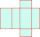Cuboid box have dimensions of 30 cm, 25 cm and 40 cm. Sketch its network and compute surface area of box.
15. Surface area of a cubeWhat is the surface area of a cube that has an edge of 3.5?
16. Cube surface areaWall of cube has content area 99 cm square. What is the surface of the cube?
17. The shopThe shop has 3 hectoliters of water. How many liter bottles is it?